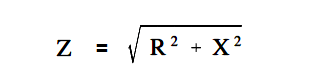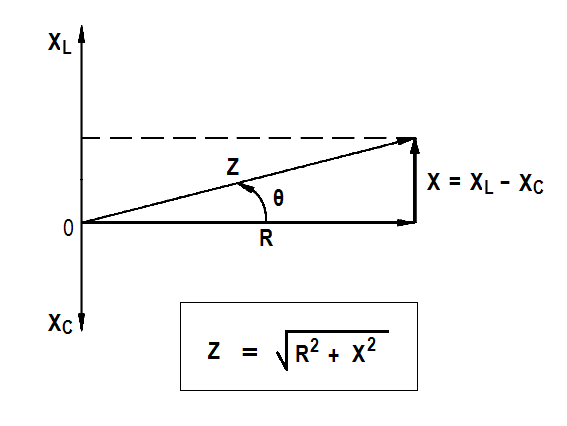# Impedance

Whenever inductive and capacitive components are used in an AC circuit, the calculation of their effects on the flow of current is important.

#### Impedance

No circuit is without some resistance, whether desired or not. Resistive and reactive components in an AC circuit oppose current flow. The total opposition to current flow in a circuit depends on its resistance, its reactance, and the phase relationships between them.

Impedance is defined as the total opposition to current flow in a circuit.

The below Equation is the mathematical representation for the magnitude of impedance in an AC circuit.where
Z = impedance (Ω)
R = resistance (Ω)
X = net reactance (Ω)

The relationship between resistance, reactance, and impedance is shown in Figure 5.Figure 5 : Relationship Between Resistance, Reactance, and Impedance

The current through a certain resistance is always in phase with the applied voltage. Resistance is shown on the zero axis. The current through an inductor lags applied voltage by 90°; inductive reactance is shown along the 90° axis. Current through a capacitor leads applied voltage by 90°; capacitive reactance is shown along the -90° axis. Net reactance in an AC circuit is the difference between inductive and capacitive reactance.

The below Equation is the mathematical representation for the calculation of net reactance when XL is greater than XC.

X = XL – XC

where

X = net reactance (Ω)
XL = inductive reactance (Ω)
XC = capacitive reactance (Ω)

The below Equation is the mathematical representation for the calculation of net reactance when XC is greater than XL.

X = XC – XL

Impedance is the vector sum of the resistance and net reactance (X) in a circuit, as shown in Figure 5. The angle θ is the phase angle and gives the phase relationship between the applied voltage and the current. Impedance in an AC circuit corresponds to the resistance of a DC circuit. The voltage drop across an AC circuit element equals the current times the impedance.

The below Equation is the mathematical representation of the voltage drop across an AC circuit.

V = IZ

where

V = voltage drop (V)
I = current (A)
Z = impedance (Ω)

The phase angle θ gives the phase relationship between current and the voltage.